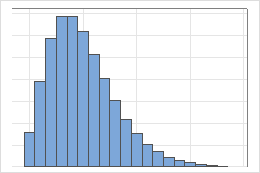# Negative binomial distribution

The negative binomial distribution is a discrete distribution that models the number of trials that are necessary to produce a specified number of events. Each trial has two possible outcomes. The event is the outcome of interest from a trial. The negative binomial distribution can also model the number of nonevents that occur before you observe the specified number of outcomes.

For example, a negative binomial distribution can model the number of times you must flip a coin to obtain five tails. Similarly, for products that are built on an assembly line, the negative binomial distribution can model the number units that are assembled before 100 defective units are produced. The following graph illustrates a negative binomial distribution with an event probability of 0.5 and 5 events needed.The negative binomial distribution is also known as the Pascal distribution.

By using this site you agree to the use of cookies for analytics and personalized content.  Read our policy